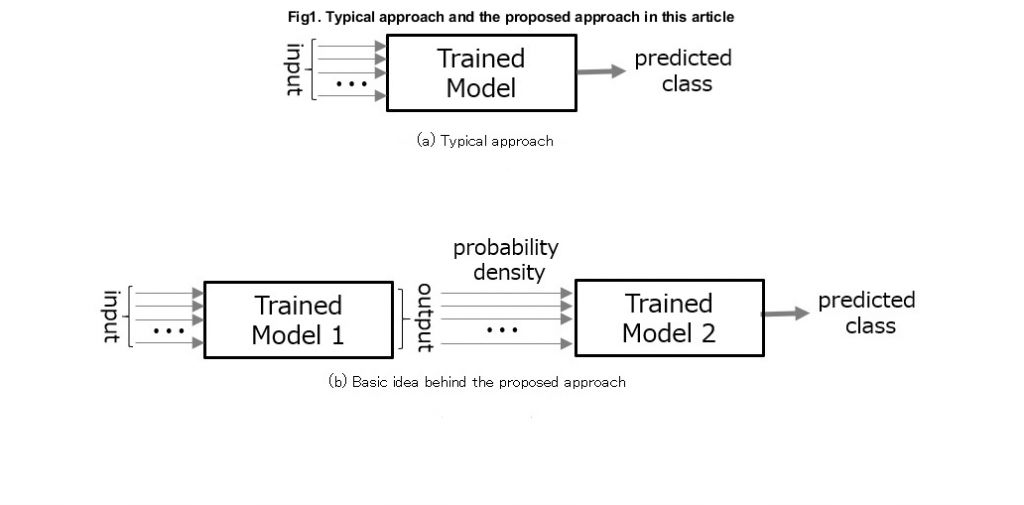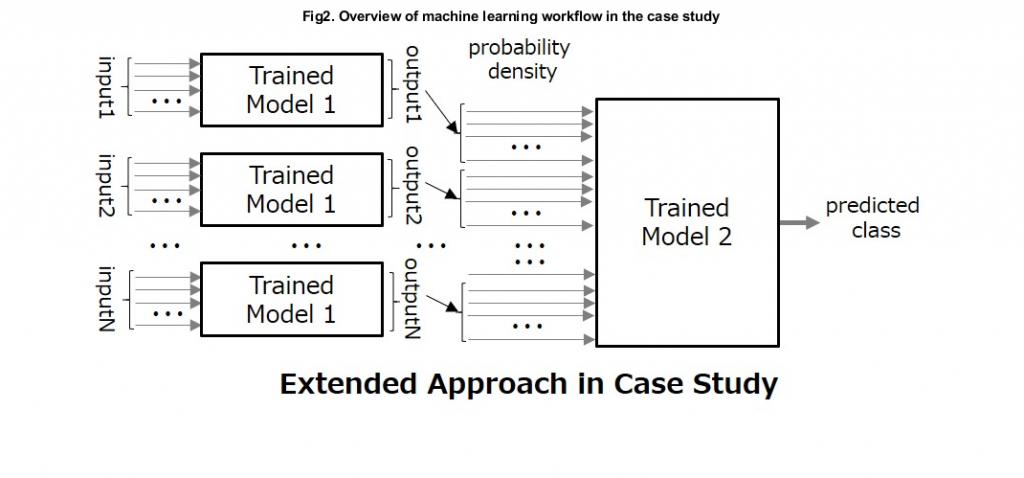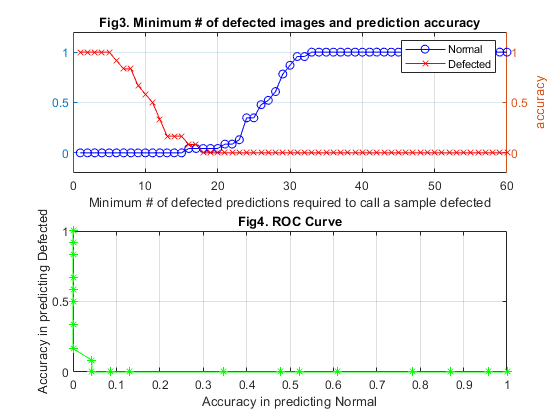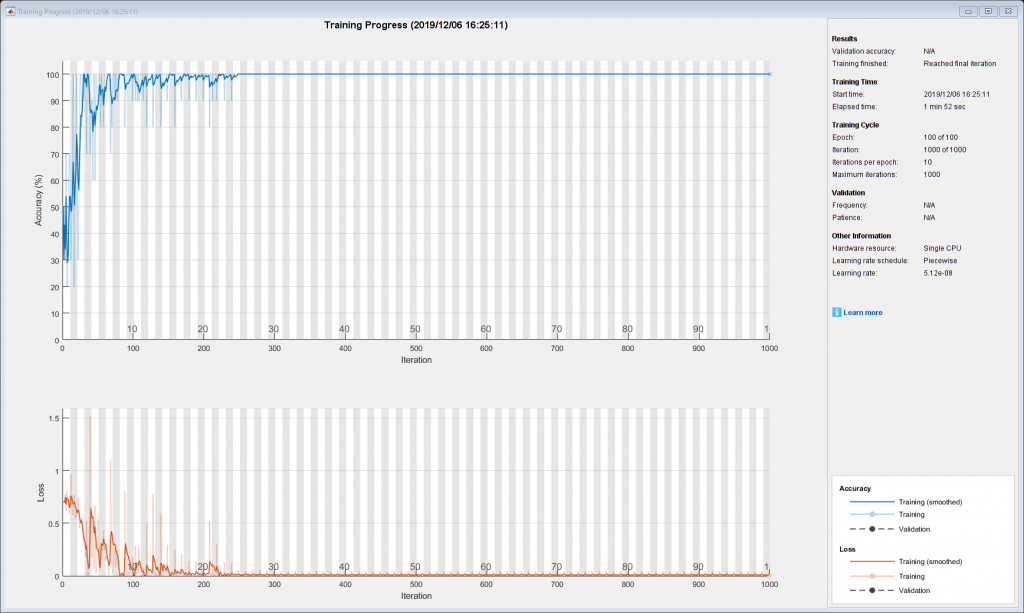# Multiple-Order Modeling for Accuracy Improvement

The following is a guest post from Mohammad Muquit here to discuss implementing multi-order modeling to improve accuracy of deep learning models. In typical classification problems, deep neural network (DNN) accuracy is measured in terms of percentage of correct class predictions against ground truths. However, DNN's final layer contains more information than just a class name, but also a probability density array containing the probability of each class in the DNN. As a result, typical approaches may ignore a significant amount of output data. In this blog we use the idea that this probability density array itself could be utilized as a set of predictors for additional modeling and look into cases where this lost information can be utilized to improve a DNN's accuracy. Fig 1. shows the basic idea behind the proposed approach.We introduce a quality assurance (QA) application as a case example here. For a given sample product going to be inspected, multiple images of that sample are used as inputs. The images are captured by rotating the sample product in front of a QA imaging system, capturing 60 images per sample. In total, 135 unique samples are used in this case study, where 55 of them had some defects and the remaining 80 samples are normal. We split this data into training and test data as follows:
 training data test data normal samples: 57 23 defected samples: 43 12 total unique samples: 100 35 total images: 6000 2100
A DNN is first generated using the training images for transfer learning with GoogleNet. The overall accuracy of the 2100 test images was 67.43%, where the accuracy for Normal and Defected images were 67.05% and 87.18%, respectively. Though it can identify individual defected images with higher accuracy, it fails to identify normal images for almost one-third of the cases. Note that these results are only at individual image-to-class level, yet we have 60 images per sample. Even when these 60 predicted results are of very low accuracy individually, collating them together for an additional model (shown below in Fig2) might result in very high accuracy, which we'll explore in the next section.Fig2: Image of the same sample are collated together to form a very long predictor array as input for a second model. This figure shows the idea of 2nd order modeling, but note that this can be further extended to multiple-order modeling.

## Approaches for multiple-order modeling

I will present 4 approaches to multiple-order modeling from simplest to most complex, all of which improves on the original accuracy of the individual image-to-class accuracy. The code to recreate these experiments and plots is available on file exchange

### Naive approach

We set a rule that if N out of the 60 images are predicted as Defected, then the sample will be called Defected. Fig3 shows how the accuracy level for Normal and Defected moves with the changes in the value of N. Fig4 shows the ROC curve.
%% Analysis of image level prediction probability data
numImg  = width(TrainDataTable)-1;
dfctIndx= TestProdLabels=='Defected'; %index of rows corresponding to Defected class
nrIndx  = ~dfctIndx; %index of rows corresponding to Normal class
Nnr     = sum(nrIndx); %Number of Normal samples
Ndf     = sum(dfctIndx); %Number of Defected samples
SA      = sum(table2array(TestDataTable) <= 0.5,2); %Number of images labeled as Defected
nAcr = zeros(numImg,1);
dAcr = zeros(numImg,1);
% For each value of N_d, the accuracy at sample-to-class level is calculated
for k = 1:numImg
rslt = SA >= k;%k represents N_d
dAcr(k) = sum(rslt == 1 & dfctIndx == 1)/Ndf;%Accuracy regarding Defected sample
nAcr(k) = sum(rslt == 0 & nrIndx == 1)/Nnr;%Accuracy regarding Normal sample
end

From the coincide of the two accuracy curves in Fig3, we can see that if we set as N as 17 or 18, then Normal and Defected samples can be detected with accuracies as low as 4.35% and 8.33%, respectively. We understand that such approach is not effective for collating outputs of a model with very low prediction accuracy against individual images.### Normal-Defected pattern as predictor for machine learning

In this second approach, we train a second model by using only category predictions of all the 60 images. So, for each individual sample, we create a 1 x 60 array of binary values, i.e., assigning a value of either 1 or 0 (i.e., Normal:1 or Defected:0). We create arrays for training samples, train a model, and then create arrays for test samples to evaluate the model. We find that the accuracy improves to more than 90%. Instead of looking at only the number of 0's or 1's, looking at how the 0's or 1's are arranged in an array is much more efficient in differentiating the samples.
% Test data table -> Binary pattern Table for test
DiscTestDataTable = double(table2array(TestDataTable(:,1:end)) > 0.5);
DiscTestDataTable = array2table(DiscTestDataTable);%Array to table conversion
For both test and training data, set the data to 1 if normal, 0 if defected. This is indicated by data that is greater than .5 in the original probability density table. So using the first sample in the test data, the conversion would look like this: Test Sample 1, images 1-20:
 0.841 0.457 0.685 0.137 0.983 0.808 0.904 0.928 0.979 0.796 0.988 0.285 0.976 0.542 0.411 0.235 0.912 0.287 0.614 0.484
Test Sample 1 with threshold of 0.5:
 1 0 1 0 1 1 1 1 1 1 1 0 1 1 0 0 1 0 1 0
We train a machine learning classifier to identify the pattern of 0's and 1's to differentiate normal and defected samples.
% Machine Learning Model Creation and Evaluation for Binary Pattern

bTM = trainClassifier(DiscTrainDataTable,numImg);
% Prediction using the Test data (Sample-to-Class level prediction)
bP = bTM.predictFcn(DiscTestDataTable);
bAcc = 100*sum(bP==TestProdLabels)/numel(bP);
disp(['Thresholded Data Accuracy:', num2str(bAcc),'%'])
Thresholded Data Accuracy: 91.4286%

### Probability distribution value as predictor for machine learning

In the previous approach, there is no guarantee that 0.5 is the best value to divide the predictors into two different classes. Therefore, using the probability density value itself (a continuous value) as predictor might be the next step for improvement. We train and evaluate models as shown in the code below. Because information loss is reduced, we see a good rise in accuracy (97.14%) in this approach compared to the previous one (91.43%).
% Machine Learning Model Creation and Evaluation for Probability Density
% Training
TM = trainClassifier(TrainDataTable,numImg);%Modeling using continuous pattern as
% Evaluation
P = TM.predictFcn(TestDataTable);% Prediction regarding the Test data (Sample-to-Class level prediction)
acc = 100*sum(P==TestProdLabels)/numel(P);
disp(['Normal Data Accuracy:',num2str(acc),'%'])

### Training a LSTM neural network

There is still one more point left, which is the coherence among the 60 probability density values obtained against 60 images, which were captured in a time-series manner. The idea is: for a given Defected sample, the defects are supposed to be visible on some of the images out of 60 images. As a result, regarding such Defected samples, probability density value indicating Defected condition should appear in a bunch. Whereas, for a given Normal sample, even if there are some probability density value indicating Defected condition by mistake, they should appear randomly caused by noise or other factors. In this section we use the probability density data for training an LSTM neural network and evaluate its accuracy. This time, the accuracy reaches 100% with a neural network trained with 100 epochs.%% Evaluation of the LSTM network
PL = classify(net,tstLstm);% Prediction on test data
lAcc = 100*sum(PL==TestProdLabels)/numel(PL);%Calculation of accuracy
disp(['LSTM Accuracy:',num2str(lAcc),'%'])
LSTM Accuracy:100%